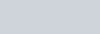The transistion from the state
Question:

The transistion from the state $\mathrm{n}=4$ to $\mathrm{n}=3$ in a hydrogen like atom results in ultraviolet radiation. Infrared radiation will be obtained in the transition from :-

1. $4 \rightarrow 2$

2. $5 \rightarrow 4$

3. $2 \rightarrow 1$

4. $3 \rightarrow 2$

Correct Option: , 2

Solution: